Statistical Physics NAT Level - 1

# Statistical Physics NAT Level - 1

Test Description

## 10 Questions MCQ Test Topic wise Tests for IIT JAM Physics | Statistical Physics NAT Level - 1

Statistical Physics NAT Level - 1 for IIT JAM 2023 is part of Topic wise Tests for IIT JAM Physics preparation. The Statistical Physics NAT Level - 1 questions and answers have been prepared according to the IIT JAM exam syllabus.The Statistical Physics NAT Level - 1 MCQs are made for IIT JAM 2023 Exam. Find important definitions, questions, notes, meanings, examples, exercises, MCQs and online tests for Statistical Physics NAT Level - 1 below.
Solutions of Statistical Physics NAT Level - 1 questions in English are available as part of our Topic wise Tests for IIT JAM Physics for IIT JAM & Statistical Physics NAT Level - 1 solutions in Hindi for Topic wise Tests for IIT JAM Physics course. Download more important topics, notes, lectures and mock test series for IIT JAM Exam by signing up for free. Attempt Statistical Physics NAT Level - 1 | 10 questions in 30 minutes | Mock test for IIT JAM preparation | Free important questions MCQ to study Topic wise Tests for IIT JAM Physics for IIT JAM Exam | Download free PDF with solutions
 1 Crore+ students have signed up on EduRev. Have you?
*Answer can only contain numeric values
Statistical Physics NAT Level - 1 - Question 1

### A system of non-interacting Fermi particles with Fermi energy EF , has the density of states proportional to √E where E is the energy of a particles. The ratio of average energy per particle at T = 0 to the Fermi energy is.

Detailed Solution for Statistical Physics NAT Level - 1 - Question 1

We know that E = U/N
and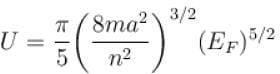and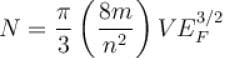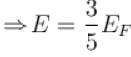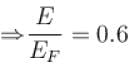*Answer can only contain numeric values
Statistical Physics NAT Level - 1 - Question 2

### In a Maxwellian gas, if Vrms is the root means square velocity, then the most probable velocity  vmp in terms of vrms is.

Detailed Solution for Statistical Physics NAT Level - 1 - Question 2

We know that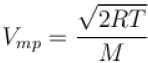and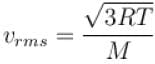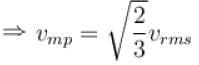vmp = 0.8165 vrms

*Answer can only contain numeric values
Statistical Physics NAT Level - 1 - Question 3

### A system has energy level E0, 2E0, 3E0,....., where the excited states are triply degenerated. Three non-interacting bosons are placed in the system. The total energy of these bosons is 5E0, the number of microstates is.

Detailed Solution for Statistical Physics NAT Level - 1 - Question 3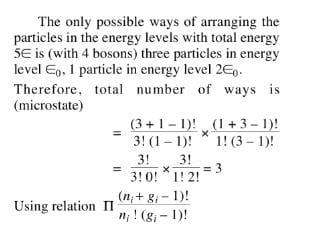*Answer can only contain numeric values
Statistical Physics NAT Level - 1 - Question 4

The total number of accessible states of non-interacting particles of spin 1/2 is given that N = 20.

Detailed Solution for Statistical Physics NAT Level - 1 - Question 4

Since the particles which exhibit odd integral spins are fermions and hence they obey Pauli’s exclusion principles. According to Pauli’s exclusion principle, not more than one particle can exist in the one quantum state. Hence, the number of assessible states N = 20.

*Answer can only contain numeric values
Statistical Physics NAT Level - 1 - Question 5

The number of coordinates in the phase space of a single particle is.

Detailed Solution for Statistical Physics NAT Level - 1 - Question 5

For a single particle, these are 3 degrees of position coordinates x, y, z and 3 degrees of momentum coordinates px, py, pz, hence total coordinates = 6.

*Answer can only contain numeric values
Statistical Physics NAT Level - 1 - Question 6

The total energy per particle of a collection of free fermions is 3eV. The Fermi energy of the system (in eV) is?

Detailed Solution for Statistical Physics NAT Level - 1 - Question 6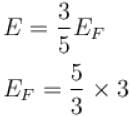= 5eV

*Answer can only contain numeric values
Statistical Physics NAT Level - 1 - Question 7

An ensemble of N three level systems with energies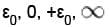in thermal equilibrium at temperature T. Let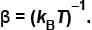If βε = 2, the probability of finding the system in level ε = 0 is given as  (1 + 2cosh 2)α . Find value of α?

Detailed Solution for Statistical Physics NAT Level - 1 - Question 7

The probability of finding the system in the energy state with energy ε may be defined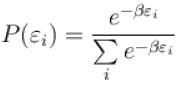But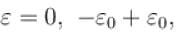hence probability of finding the sytem with ε = 0 is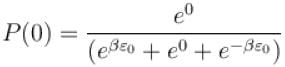In question, βε0 = 2 so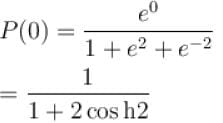P(0) = (1 + 2cosh 2)-1
⇒ α = 0.

*Answer can only contain numeric values
Statistical Physics NAT Level - 1 - Question 8

The dimension of phase space of ten rigid diatomic molecule is

Detailed Solution for Statistical Physics NAT Level - 1 - Question 8

For a rigid diatomic molecule, there is no rotation possible along the inter-nuclear axis. That remove one angle from 3 Euler angles needed to describe the orientation of the molecules, and hence also removes an angular momentum component. That leaves 4 angular degrees of freedom plus 3 coordinate and 3 momenta for the center of mass motions.
∴ One diatomic molecule has phase space of dimension 10, so the number ofphase space for10 diatomic molecule = 10 ×10 = 100.

*Answer can only contain numeric values
Statistical Physics NAT Level - 1 - Question 9

For a particle moving in a circle of fixed radius with constant speed, dimension of phase space is.

Detailed Solution for Statistical Physics NAT Level - 1 - Question 9

For a 2D moving in a plane as in case of a circular motion, we have two degrees of freedom for the q i.e. for x – y plane, q1 = x and q2 = y, and two degrees of freedom for the velocity given by ux and vy and thus px and py.
⇒ dimension of phase space = 2 + 2 = 4.

*Answer can only contain numeric values
Statistical Physics NAT Level - 1 - Question 10

A system of N non-interacting classical point particles is constrained to move on the two-dimensional surface of a sphere. The internal energy of the system is in units of NkBT?

Detailed Solution for Statistical Physics NAT Level - 1 - Question 10

Since the particle is moving on two dimensional surface, its degree of freedom = 2.
Hence, for N non-interacting particles total degree of freedom = 2N
The energy per degree of freedom = 1/2 kBT
Hence, total energy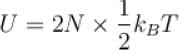U = NkBT

## Topic wise Tests for IIT JAM Physics

217 tests
 Use Code STAYHOME200 and get INR 200 additional OFF Use Coupon Code
Information about Statistical Physics NAT Level - 1 Page
In this test you can find the Exam questions for Statistical Physics NAT Level - 1 solved & explained in the simplest way possible. Besides giving Questions and answers for Statistical Physics NAT Level - 1, EduRev gives you an ample number of Online tests for practice

217 tests